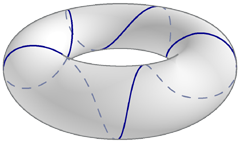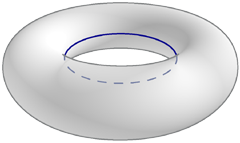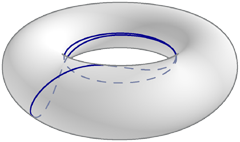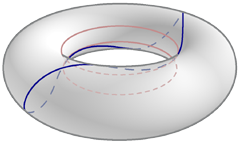]> The Geodesics of the Torus

# The Geodesics of the Torus

Here be monsters, or at least varmints

## The Geodesic Equation

Part of our computation of the torus's curvature was the computation of the Christoffel symbols of the second kind. They also appear in the geodesic equation

$x ‥ a + Γ bc a x ˙ b x ˙ c = 0$

Plugging in our results, we get a pair of differential equations.

$u ‥ − 2 a sin v c + a cos v u ˙ v ˙ = 0 v ‥ + 1 a sin v c + a cos v u ˙ 2 = 0$

Using the substitution $w = c + a cos v$ and integrating (with a trick or two) gives a solution in terms of $u ˙$ and $v ˙$ :

$u ˙ = k c + a cos v 2 v ˙ = ± − k 2 a 2 c + a cos v 2 + l$

where k and l are constants of integration.

We'd like to integrate these equations to get u as a function of v or vice versa. However, the constant of integration l throws a monkey wrench into the works, and we can't integrate them. That's not the only bad news: most (k,l) pairs don't yield geodesics, and without a way to relate one constant to the other, we have no way to decide whether a given pair yields a geodesic or not. (Thought question: why are there two constants of integration, not one? Only one parameter—a direction—is required to choose a unique geodesic through a given point, not counting reversal of direction along the chosen geodesic.)

The same problem dashes our hopes of finding a unit speed parameterization. We've hit a dead end; does the Clairaut parameterization help?

## The Clairaut Parameterization

The Clairaut parameterization of a torus treats it as a surface of revolution. From it, we obtain a formula for $du dv$ .

$du dv = ± ah c + a cos v c + a cos v 2 − h 2$

Using this difference equation (which, like the solution to the geodesic equation, can't be integrated), we can find the families of geodesics on the torus by varying the parameter h, which is the "slant" of a geodesic. It governs the angle φ at which a geodesic crosses parallels.

$h = c + a cos v sin φ$

For a given geodesic h is constant, which means that as the geodesic passes through regions of different curvature, the angle it makes with parallels changes to compensate. For the torus, this means that as v changes, so must φ.

Returning to the formula for $du dv$, the term under the radical, $c + a cos v 2 − h 2$, must be positive. This bounds h: $h ≤ c + a$. Armed with this fact, we can now categorize the geodesics of the torus by their h value.

## The Five Families of Geodesics

[Notes: in this section, we consider only the absolute value of h, not its sign. Changing the sign of h yields a mirror image of the original geodesic. Also, to be precise, this section considers pregeodesics, since we're not providing unit speed parameterizations.]

$h = 0$. These geodesics are simple: as v changes u doesn't, so these are the meridians.[An intuitive way to see that meridians are geodesics is to realize that the torus has a mirror symmetry through meridians. Anything that would push the geodesic off a meridian in one direction has a balancing mirror image counterpart, so a geodesic that starts on a meridian cannot leave it. A similar argument can be made for both the inner and outer equators, hence they must be geodesics also.]

$0 < h < c − a$. The geodesics now diverge from the meridians, and loop around the torus in the v direction, crossing both the inner and outer equators. Since a geodesic can pass through any point on the surface, we call these unbounded geodesics.Note in the figure above the difference in slant of the geodesic as it crosses the inner and outer equators. This is a consequence of the definition of h.

The closer h is to zero, the more tightly wound are the geodesic's coils. As h increases, the coils spread out and the angles at which the geodesic crosses the equators decrease. Since the crossing angle at the inner equator is always less than the crossing angle at the outer equator, the former will reach zero sooner. This is the next case.

$h = c − a$. When h reaches this value, the only angle a geodesic can make with the inner equator is zero. Hence a geodesic starting on the inner equator must remain on it, so the inner equator is a geodesic.The geodesics with this h value that don't start on the inner equator are asymptotic to it. They spiral ever closer to the inner equator without touching it. (This is hard to illustrate without the ability to draw infinitely thin lines. Use your imagination.) This geodesic is unique barring rotation around the z axis and reflection through the xy plane.These geodesics are the edge case of the next family, the bounded geodesics.

$c − a < h < c + a$. Things start getting interesting here. A consequence of the Clairaut parameterization is that a geodesic can't leave the region where $c + a cos v 2 ≥ h 2$ . This is trivially satisfied for the preceding cases where $h ≤ c − a$ , but when $h > c − a$ (as it now is), something surprising happens: there's a region of the torus which a geodesic can't reach. Instead, geodesics are restricted to the outer region of the torus between two parallels, known as barrier curves. (The pink curves in the illustration.) These geodesics cross the outer equator, but when they touch a barrier curve they reverse v-direction and bounce off it. We call these the bounded geodesics.When $h = c − a$ the barrier curves coincide at the inner equator. As $h$ increases, the barrier curves sweep out from the inner equator, and converge on the outer equator as $h → c + a$ .

$h = c + a$. The last geodesic is the limit of the bounded geodesics, the outer equator.When $h > c + a$, there are no real solutions to the formula for $du dv$.

This completes our survey of the families of a torus's geodesics. To summarize:

Slant $h$ Geodesics
0 meridians
$0 < h < c - a$ unbounded geodesics, which alternately cross inner and outer equators
$c - a$ the inner equator, and geodesics asymptotic to it
$c − a < h < c + a$ bounded geodesics, which cross the outer equator but bounce off barrier curves
$c + a$ the outer equator

## A Gallery of Geodesics

The geodesics in the illustrations above were carefully chosen. Most aren't as photogenic; they're aperiodic and cover either the entire torus (for unbounded geodesics) or the entire region between the barrier curves (for bounded geodesics). If we define a geodesic's period as the number of times it circles the z axis before returning to its starting point, then the photogenic geodesics are the unbounded geodesics of period 1, and bounded geodesics of period 1 or 2.

The unbounded geodesics of period 1 cross each equator n times ($n ≥ 1$).

The interesting bounded geodesics fall into two groups. Those of period 1 do not self-intersect. (For bounded geodesics, n denotes how many times the geodesic touches each barrier curve.)n = 1

The geodesics of period 2 intersect themselves n times (n odd, of course).

For bounded geodesics the allowed values of n depend on the ratio c/a. Unbounded geodesics are not affected by c/a.

## Open Questions

### The influence of c/a on bounded geodesics

The kinds of bounded geodesics one can find on a particular torus are determined not only by h, but also by the ratio c/a. For instance, given a torus with c/a=3/1, there is no period 1 bounded geodesic which touches each barrier curve more than once. Yet for a torus with c/a=8/1, there is a period 1 bounded geodesic which touches each barrier curve twice, and another which touches each barrier curve three times.

This raises a question: what is the range of c/a of the tori that contain bounded period 1 geodesics which touch each barrier curve exactly n times, as a function of n? How about for different periods? There is no analytic apparatus I know of with which we can approach the problem. Calculation appears to be the only way to go.

Note that this restriction applies only to bounded geodesics. There is no corresponding restriction for unbounded geodesics; by choosing an appropriate h, one can find a period 1 geodesic which crosses both equators as often as one pleases.

### Questions about critical values of h

Another open question concerns the values of h which yield crowd-pleasing geodesics with periods 1 and 2. As c/a changes, so does the value of h which yields a particular pleasing geodesic (say, a period 1 geodesic which crosses both equators three times). Is there a simple relation between these two quantities?

A similar question exists for values of h for a given c/a. Define hp as the value of h which yields a period 1 geodesic which crosses both equators p times. As p increases, at what rate does hp converge to 0? Is this governed by a simple rule? What about period 2 bounded geodesics?

## Lessons Learned

About the illustrations. I've attempted to make this page's illustrations as accurate as possible, but there's an inevitable tradeoff between illustrative power and accuracy. If you're curious, you can read about how I created them.

Last updated 3 March 2005
http://www.rdrop.com/~half/math/torus/geodesics.xhtml
All contents released into the public domain by Mark L. Irons